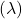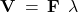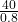## The speed of a wave is 40 m/s. If the wavelength is 80 centimeters, what is the frequency of the wave? SOLVE IT COMPLETELY

Question

The speed of a wave is 40 m/s. If the wavelength is 80 centimeters, what is the frequency of the wave?

SOLVE IT COMPLETELY

in progress 0
3 months 2021-08-18T06:41:43+00:00 1 Answers 0 views 0

1. ## Question:-

The speed of a wave is 40 m/s. If the wavelength is 80 centimeters, what is the frequency of the wave ?

### Given:-

Velocity (V) = 40 m/s

Wavelength= 80 cm = 0.8 m

### To Find:-

The frequency (F) of the wave.

## Solution:-

We know,40 = F × 0.8

F =F = 50